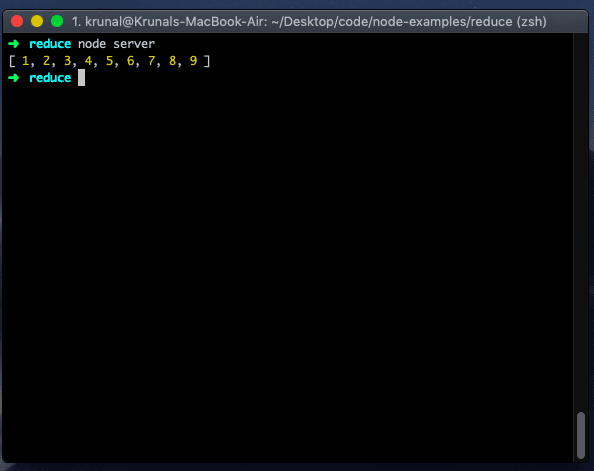# JavaScript Array reduce() Method

JavaScript array reduce() function is “used to execute a reducer function on each element of the array and returns a single output value”.

### Syntax

``````array.reduce(function(total, currentValue, currentIndex, arr), initialValue)

# or

array.reduce(callback[, initialValue]);``````

### Parameters

1. function(total, currentValue, index, arr): Each array element is a required parameter. It contains four parameters which are listed below:
1. total: It is the required parameter used to specify an initialValue or the previously returned value of a function.
2. currentValue: It is the needed parameter to determine a current element’s value.
3. currentIndex: The optional parameter is used to specify an array index of the current element.
4. arr: It is the optional parameter to determine an array object to which the current element belongs.
2. initialValue: The optional parameter specifies the value to be passed to the function as an initial value.

### Return Value

Each time a function is called, its return value becomes the first argument for the next time it’s called. So if we have to find a sum of all the numbers in the array, we could use the javascript array reduce() function.

Note that we can also pass the third argument into Reduce. This value then becomes the starting value for our accumulator. So, it is also called the initial value.

By default, the starting value of an accumulator is the first element of the array we pass in.

## Example 1: How to Use the array reduce() function

The reduce() function executes the provided function for each value of an array from left to right. The return value of a function is stored in an accumulator.

``````data = [2, 4, 6, 8, 10]

const reducer = (accumulator, currentValue, currentIndex, array)
=> accumulator + currentValue

result = data.reduce(reducer)

console.log(result)``````

The reducer() function takes four parameters.

Let’s see a descriptive table showing what happens on each iteration.

callback iteration accumulator currentValue currentIndex array return value
first, call 2 4 1 [2, 4, 6, 8, 10] 6
second call 6 6 2 [2, 4, 6, 8, 10] 12
third call 12 8 3 [2, 4, 6, 8, 10] 20
fourth call 20 10 4 [2, 4, 6, 8, 10] 30

The value returned by the reduce() would be that of the last callback invocation 30.

## Example 2: Passing no initial value to the reduce() Function

If you don’t pass the initial value, the reducer will assume the first item in your array as your initial value. This worked fine in some examples because we added the list of numbers.

``````data = [11, 21, 19, 18, 46]

const reducer = (accumulator, currentValue) => accumulator + currentValue

result = data.reduce(reducer)

console.log(result)``````

Output

``115``

## Example 3: Passing an initial value to the reduce() function

If we pass the initial value as an argument, it will position itself as the first value and then reduce it.

``````data = [11, 21, 19, 18, 46]

const reducer = (accumulator, currentValue) => accumulator + currentValue

result = data.reduce(reducer, 29)

console.log(result)``````

Output

``144``

## Example 4: Flattening arrays using Javascript reduce method

To flatten an array in JavaScript, use the array.reduce() method. We can use the reduce() function to flatten the nested amounts into a single array. We set an initial value to the empty array and then concatenate the current value to the total.

``````const data = [[1, 2, 3], [4, 5, 6], [7, 8, 9]];

const flatValues = data.reduce((total, value) => {
}, []);

console.log(flatValues);``````

Output## Example 5: Finding repeated elements in an array

Let’s say you have a collection of items and want to know how many of each item is in the collection.

In this scenario, the Javascript reduce() method can help us.

``````const pounds = [11, 21, 16, 19, 46, 29, 46, 19, 21];

const count = pounds.reduce( (data, pound) => {
data[pound] = (data[pound] || 0) + 1 ;
return data;
} , {})

console.log(count);``````

Output

``{ '11': 1, '16': 1, '19': 2, '21': 2, '29': 1, '46': 2 }``

## Example 6: Grouping objects by a property

We can group objects by their property using the array.reduce() method.

``````let student = [
{ name: 'Rick', enrollment: 60 },
{ name: 'Beth', enrollment: 40 },
{ name: 'Jerry', enrollment: 40 }
];

function groupBy(objectArray, property) {
return objectArray.reduce(function (accumulator, obj) {
let key = obj[property]
if (!accumulator[key]) {
accumulator[key] = []
}
accumulator[key].push(obj)
return accumulator
}, {})
}

let groupedStudent = groupBy(student, 'enrollment')
console.log(groupedStudent)``````

Output

``````{
'40': [
{ name: 'Beth', enrollment: 40 },
{ name: 'Jerry', enrollment: 40 }
],
'60': [ { name: 'Rick', enrollment: 60 } ]
}``````

## Browser compatibility

1. Google Chrome 3 and above
2. Microsoft Edge 12 and above
3. Mozilla Firefox 3.0 and above
4. Safari 5 and above
5. Opera 10.5 and above

That’s it.

This site uses Akismet to reduce spam. Learn how your comment data is processed.